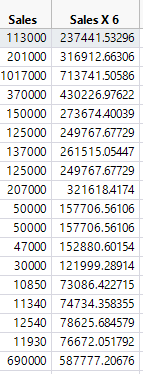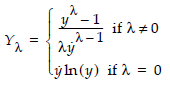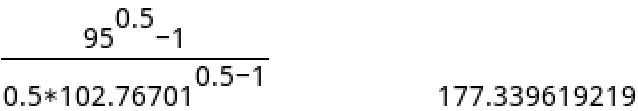Choose Language Hide Translation Bar
Highlighted

## Box Cox Y Transformation - Show untransformed values in Prediction Profiler

Hello,

after applying a Box Cox Y Transformation to a response, the Prediction Profiler - as far as I understand - only shows the transformed response. So also the desirabilities have to be based on these transformed values. The prediction formula only leads to the transformed response as well. Is there any approach to treat this? I would appreciate to work with retransformed values in the Prediction Profiler.

Regards,

Ben

1 ACCEPTED SOLUTION

Accepted Solutions
Highlighted

## Re: Box Cox Y Transformation - Show untransformed values in Prediction Profiler

Using a transformed response or predictor / factor / explanatory variable requires a shift in thinking. All of the analysis is in terms of the transformed variable. Most scientests today, for example, would not transform pH back to hydronium activity.

The nature of the transform might make it easier to back transform the response. For example, if the transform is square root Y, then simply square the prediction. We usually select a value for the Box-Cox transform that corresponds to a physical reality as natural dimensions and relationships often work that way. You can generally think of the value as a power, though it is not exactly the same. So a parameter value of -0.5 would correspond to reciprocal square root.

Once you identify the nature of the transform, you might be able to use a transform in the Fit Model dialog instead of using the Box-Cox transform. JMP will then profile the response in terms of the original response. Otherwise, uou can save the model for the transformed response from Fit Least Squares by clicking the red triangle and selecting Save Columns > Prediction Formula. You can then select the column, select Cols > Formula, and apply the inverse function. After saving the changes to the formula, select Graph > Profiler, select the modified prediction formula, and click OK.

Learn it once, use it forever!
4 REPLIES 4
Highlighted

## Re: Box Cox Y Transformation - Show untransformed values in Prediction Profiler

Using a transformed response or predictor / factor / explanatory variable requires a shift in thinking. All of the analysis is in terms of the transformed variable. Most scientests today, for example, would not transform pH back to hydronium activity.

The nature of the transform might make it easier to back transform the response. For example, if the transform is square root Y, then simply square the prediction. We usually select a value for the Box-Cox transform that corresponds to a physical reality as natural dimensions and relationships often work that way. You can generally think of the value as a power, though it is not exactly the same. So a parameter value of -0.5 would correspond to reciprocal square root.

Once you identify the nature of the transform, you might be able to use a transform in the Fit Model dialog instead of using the Box-Cox transform. JMP will then profile the response in terms of the original response. Otherwise, uou can save the model for the transformed response from Fit Least Squares by clicking the red triangle and selecting Save Columns > Prediction Formula. You can then select the column, select Cols > Formula, and apply the inverse function. After saving the changes to the formula, select Graph > Profiler, select the modified prediction formula, and click OK.

Learn it once, use it forever!
Highlighted

## Re: Box Cox Y Transformation - Show untransformed values in Prediction Profiler

Thank you very much for your explanation an the two helpful approaches.
Highlighted

## Re: Box Cox Y Transformation - Show untransformed values in Prediction Profiler

My apologies for the probably very bad question. I did a Box Cox transformation of my data but when I saved the transformed data, it wasn't what I had expected.

The lambda associated with my Box Cox transformation was 0.5. The first handful of transformed values are attached. The first untransformed value was 113,000 while the transformed value was 237,441.53296. This does not correspond to any Box Cox transformation formula I've seen on the web including that on the JMP website. Could someone please clarify exactly what the transformation formula is?Highlighted

## Re: Box Cox Y Transformation - Show untransformed values in Prediction Profiler

Here is the definition of the calculation of the transformation used by JMP:Note that the denominator uses the geometric mean of y. I cannot check your result because I cannot obtain the geometric mean for your untransformed data.

I applied the Box-Cox transform to the :weight variable in Big Class using lambda = 0.5 and got 95 => 177.3396219 in the data table. I get the same result when I compute it directly:Learn it once, use it forever!
Article Labels

There are no labels assigned to this post.